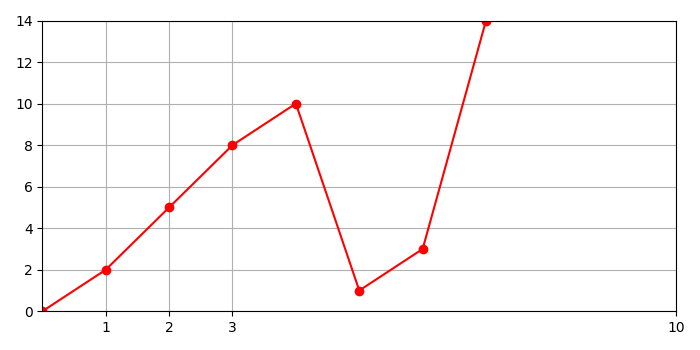# Define the size of a grid on a plot using Matplotlib

To define the size of a grid on a plot, we can take the following steps −

• Create a new figure or activate an existing figure using figure() method.

• Add an axes to the figure as a part of a subplot arrangement.

• Plot a curve with an input list.

• Make x and y margins 0.

• To set X-grids, we can pass input ticks points.

• To lay out the grid lines in current line style, use grid(True) method.

• To display the figure, use show() method.

## Example

from matplotlib import pyplot as plt
plt.rcParams["figure.figsize"] = [7.00, 3.50]
plt.rcParams["figure.autolayout"] = True
fig = plt.figure()
plt.show()